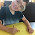## Elementary Studies

### Odd and Even numbers

Even numbers: Even numbers are the numbers that are divisible by 2. For example, 8 can be divided by 2, so 8 is an even number.

Odd numbers: Odd numbers are the numbers that are not divisible by 2. For example, 9 cannot be divided by 2, so 9 is an odd number.

This definition works well with the kids who are familiar with multiplication and division. As this concept is introduced to first graders or second graders where a majority of kids are not familiar with Multiplication and Division, so we should teach this in a different way.

Even numbers: A number is even if it can be divided into groups of 2 and have no leftovers. For example, 8 can be divided into 4 groups of 2 and there is no leftover, so 8 is an even number.

Odd numbers: A number is odd if it can be divided into groups of 2 and have 1 leftover. For example, when dividing 9 into groups of 2 we can make 4 groups of 2 and there is still 1 leftover, so 9 is an odd number.

• An even number ends in 0, 2, 4, 6, or 8.
• An odd number ends in 1, 3, 5, 7, or 9.

For example, 239 is an odd number as it has 9 at ones place. Similarly, 238 is even number as it has 8 at ones place.

Have a look at my Odd and Even numbers packet, which includes the no prep worksheets and activities to learn and reinforce the concept of Odd and Even numbers.

Here is the list of resources included in this even and odd numbers packet:
1. Cut and paste activities.
2. Even and Odd coloring activity.
3. Ice cream scoop activity.
4. Making a paper chain - craft activity.
5. Fun worksheets.
You can buy this Even and Odd numbers worksheets pack from HERE.

#### Literature to go with this topic:

Even Steven and Odd Todd by Kathryn Cristaldi.

This is a funny book about two cousins Steven and Todd. Even Steven likes everything even and Odd Todd likes everything odd. A great read for first and second graders. Check this out on Amazon HERE.

Happy Teaching!

Shimps

1.2.